# Quasi-equivalent representations

(diff) ← Older revision | Latest revision (diff) | Newer revision → (diff)
Two unitary representations (cf. Unitary representation)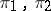of a group(or symmetric representations of a symmetric algebra) in Hilbert spacesand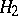, respectively, satisfying one of the following four equivalent conditions: 1) there exist unitarily-equivalent representations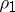andsuch thatis a multiple ofand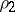is a multiple of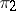; 2) the non-zero subrepresentations ofare not disjoint from, and the non-zero subrepresentations ofare not disjoint from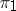; 3)is unitarily equivalent to a subrepresentation of some multiple representationofthat has unit central support; or 4) there exists an isomorphismof the von Neumann algebra generated by the set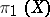onto the von Neumann algebra generated by the setsuch thatfor all. Unitarily-equivalent representations are quasi-equivalent representations; irreducible quasi-equivalent representations (cf. Irreducible representation) are unitarily equivalent. Ifandare quasi-equivalent representations andis a factor representation, then so is; a factor representation and a non-zero subrepresentation of it are quasi-equivalent representations; two factor representations are either disjoint or quasi-equivalent. The notion of quasi-equivalent representations leads to that of a quasi-dual object and a quasi-spectrum for locally compact groups and symmetric algebras, respectively.